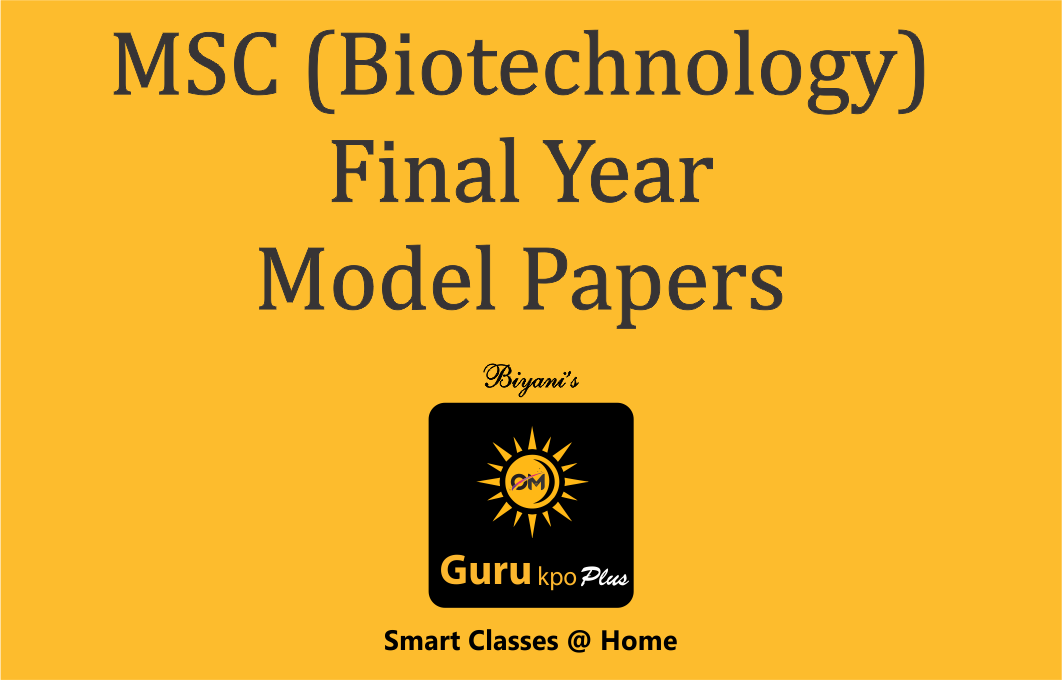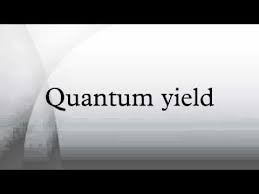## MSC (Biotechnology) Final Year Model Papers

#### Top Model Papers#### MCA Fifth Semester Model Papers#### MCA Third Semester Model Papers#### MCA First Semester Model Papers#### MSC (Biotechnology) Previous Year Model Papers#### MSC (Biotechnology) Final Year Model PapersEinstein or photochemical equivalence law is applicable only to the primary photochemical process or in other words as a result of primary absorption of one quantum, only one molecule undergoes dissociation and the products enter no further reaction. It means the ratio of number of molecules excited and number of quanta absorbed is 1:1.
In actual practice in most of the cases an activated molecule initiates a series of thermal reactions called secondary reactions as in the cases of chain reactions like between H2 and Br2 and H2 and Cl2 etc. As a result in such reactions many reactant molecules may undergo chemical change by absorbing one quantum only and the ratio deviates from 1:1.
To describe the deviation from 1:1 the term quantum efficiency or quantum yield (ϕ) was introduced . This can be defined as follows:
“It is the number of molecules which undergo chemical change per quantum of absorbed energy” or in other words the ratio of the number of molecules reacting in a given time and the number of quanta absorbed in the same time is called quantum efficiency or quantum yield .

?= No. of molecules reacting in a given time
___________________________________________
No. of quanta or photons absorbed in the same time
or
?= No. of moles reacting in a given time
___________________________________
No. of einsteins absorbed in the same time
or
?= Rate of chemical reaction
_______________________
No. of einsteins absorbed
or =
Rate of chemical reaction
____________________
No. of einsteins of light
or
?= -d[A]/dt
_____________
Energy of absorbed light

The concept of quantum yield can be extended to any physical or chemical reaction following light absorption. The quantum yield is dependent on the light intensity. The value of quantum yield vary from very low of 0.04 to very high value of the order of 106.

Experimental Determination of Quantum Yield or Efficiency

We have seen in the last section that quantum yield or efficiency is defined as
?=Rate of chemical reaction
Energy of absorbed light
Thus , in order to determine the value of quantum yield of photochemical reaction it is essential to known (i) Number of moles reacting and (ii) number of Einstien absorbed . Number of molecules reacting in given time can be determined by the chemical kinetics , since it is nothing but is the rate of reaction.

Author: Pooja Gupta